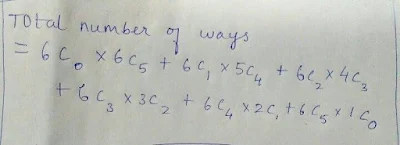New Student User - Use Code HELLO

# Maths Quiz For IBPS PO: Part - 5

### Question 1:

A survey was conducted on 100 children about their liking for 3 fruits i.e. Apple , Banana and Cherry .It was found that 20 children did not like any of these fruits. There were 30 children who liked apple , 30 liked banana and 40 liked cherry. Also, the number of children who liked all the three fruits were 5 .Find the number of children who liked exactly two fruits.
a)10
b) 20
c) 12
d) cannot be determined

### Question 2:

From among 6 couples , a committee of five members is to be formed. If the selected committee has no couple , then in how many ways can the committee be formed ?
a) 6
b) 792
c)40
d) 192

### Question 3:

Arun invested a sum of money at a certain rate of simple interest for a period of four years . Had he invested the same sum for a period of six years the total interest earned by him would have been fifty per cent more than the earlier interest amount . What was the rate of interest per annum ?
a) 4
b) 8
c)5
d) cannot be determined
e) no one of these

Directions (4-5): Each of the questions below consists of a question and two statements numbered I and II given below it. You have to decide whether the data provided in the statements are sufficient to answer the question. Read both the statements and give answer-
a)If the data in both statements I and II together are not sufficient to answer the question.
b) If the data in both the statement I and II together are necessary to answer the question.
c) If either of the data in statement I alone or in statement II alone are sufficient to answer the question.
d) If the data in statement I alone are sufficient to answer the question, while the data in statement II alone are not sufficient to answer the question
e) If the data in statement II alone are sufficient to answer the question, while the data in statement I alone are not sufficient to answer the question.

### Question 4:

55% of the employees in a certain office have a tv and a refrigerator . What percentage of employees in the office have a tv, but no refrigerator ?
(i) 65% of the employees have a tv.
(ii) 75% of the employees have a refrigerator .

### Question 5:

There are 180 blue and red balls in a cylinder and a square box together. What is the number of blue balls in the square box ?
(i) There are70 balls in the square box
(ii) There are30 blue balls in the cylinder

## Solution

#### 1) a

The given data can be represented through a venn diagram ,as shown below:IA =Number of children who liked only apple
IB = Number of children who liked only banana
IC = Number of children who liked only cherry
IIAB = Number of children who liked apple and banana only
IIBC= Number of children who liked banana and cherry only
IIAC= Number of children who liked apple and cherry only
III= Number of children who liked all the three fruits
Where , U = total number of children surveyed = 100
A=Number of children who liked apple = (IA + IIAB + IIAC + III ) = 30 ……. (i)
B= Number of children who liked banana =( IB + IIBC + IIAB + III ) =30 …… (ii)
C = Number of children who liked cherry = (IC + IIBC+ IIAC +III ) = 40 …….. (iii)
Let,
Y= number of children who did not like any of these fruits = 20
X=Number of children who like at least one of the fruits = U - Y =80
S= sum of all the three sets = A+B+C = 30+30+40 = 100
THEN , number of children who liked exactly one fruit (either apple or banana or cherry ) = I = IA + IB + IC
number of children who liked two fruits = IIBC + IIAB + IIAC = II
X = I+ II+III
Looking at (i) (ii) and (iii) and rearranging the terms we get
S = IA + IB + IC + IIAB + IIAC + IIBC + IIAB + IIBC+ IIAC + III + III+ III
=I + 2 II + 3 III
So S-X = (I + 2II + 3III ) – (I + II + III)
= II + 2 III
Putting the values in above result we get 100 – 80 = II + 2(5)
So number of children who liked exactly two fruits = II = 10

#### 2) d

(representing cases where no males are selected ; 1 male is selected while his partner is not ; two males are selected while their partners are not ; and so on , through to ; no female is selected ) = 192 Hence (d)Alternatively , the number of ways the committee can be formed = 6c5 * 25 = 192 , hence (d)

#### 3) d

cannot be determined . (d)

#### 4) c

(c) Using any one statement you can find the answer .

#### 5) a

total number of balls = 180
Statement 1 : number of balls in the square box is 70 .threfore , number of balls in the cylinder is 110 but the breakup as blue or red is not known .
Statement II : number of blue balls I nthe cylinder is 30 .But the total number of blue balls is not known . Hence , number of blue balls in the square box cannot be found out.
After combining statements I and II too answer cannot be found . Hence (a)